# Red Black Tree (RB-Tree) Using C++

A red–black tree is a special type of binary tree, used in computer science to organize pieces of comparable data, such as text fragments or numbers. In addition to the requirements imposed on a binary search tree the following must be satisfied by a red–black tree:
1. A node is either red or black.
2. The root is black. (This rule is sometimes omitted. Since the root can always be changed from red to black, but not necessarily vice versa, this rule has little effect on analysis).
3. All leaves (NIL) are black. All leaves are of the same color as the root.
4. Every red node must have two black child nodes, and therefore it must have a black parent.
5. Every path from a given node to any of its descendant NIL nodes contains the same number of black nodes.

// C++ Program to implement Red Black Tree(RB-Tree).

```#include<iostream>

using namespace std;

struct node
{
int key;
node *parent;
char color;
node *left;
node *right;
};
class RBtree
{
node *root;
node *q;
public :
RBtree()
{
q=NULL;
root=NULL;
}
void insert();
void insertfix(node *);
void leftrotate(node *);
void rightrotate(node *);
void del();
node* successor(node *);
void delfix(node *);
void disp();
void display( node *);
void search();
};
void RBtree::insert()
{
int z,i=0;
cout<<"\nEnter key of the node to be inserted: ";
cin>>z;
node *p,*q;
node *t=new node;
t->key=z;
t->left=NULL;
t->right=NULL;
t->color='r';
p=root;
q=NULL;
if(root==NULL)
{
root=t;
t->parent=NULL;
}
else
{
while(p!=NULL)
{
q=p;
if(p->key<t->key)
p=p->right;
else
p=p->left;
}
t->parent=q;
if(q->key<t->key)
q->right=t;
else
q->left=t;
}
insertfix(t);
}
void RBtree::insertfix(node *t)
{
node *u;
if(root==t)
{
t->color='b';
return;
}
while(t->parent!=NULL&&t->parent->color=='r')
{
node *g=t->parent->parent;
if(g->left==t->parent)
{
if(g->right!=NULL)
{
u=g->right;
if(u->color=='r')
{
t->parent->color='b';
u->color='b';
g->color='r';
t=g;
}
}
else
{
if(t->parent->right==t)
{
t=t->parent;
leftrotate(t);
}
t->parent->color='b';
g->color='r';
rightrotate(g);
}
}
else
{
if(g->left!=NULL)
{
u=g->left;
if(u->color=='r')
{
t->parent->color='b';
u->color='b';
g->color='r';
t=g;
}
}
else
{
if(t->parent->left==t)
{
t=t->parent;
rightrotate(t);
}
t->parent->color='b';
g->color='r';
leftrotate(g);
}
}
root->color='b';
}
}

void RBtree::del()
{
if(root==NULL)
{
cout<<"\nEmpty Tree." ;
return ;
}
int x;
cout<<"\nEnter the key of the node to be deleted: ";
cin>>x;
node *p;
p=root;
node *y=NULL;
node *q=NULL;
int found=0;
while(p!=NULL&&found==0)
{
if(p->key==x)
found=1;
if(found==0)
{
if(p->key<x)
p=p->right;
else
p=p->left;
}
}
if(found==0)
{
return ;
}
else
{
cout<<"\nDeleted Element: "<<p->key;
cout<<"\nColour: ";
if(p->color=='b')
cout<<"Black\n";
else
cout<<"Red\n";

if(p->parent!=NULL)
cout<<"\nParent: "<<p->parent->key;
else
cout<<"\nThere is no parent of the node.  ";
if(p->right!=NULL)
cout<<"\nRight Child: "<<p->right->key;
else
cout<<"\nThere is no right child of the node.  ";
if(p->left!=NULL)
cout<<"\nLeft Child: "<<p->left->key;
else
cout<<"\nThere is no left child of the node.  ";
cout<<"\nNode Deleted.";
if(p->left==NULL||p->right==NULL)
y=p;
else
y=successor(p);
if(y->left!=NULL)
q=y->left;
else
{
if(y->right!=NULL)
q=y->right;
else
q=NULL;
}
if(q!=NULL)
q->parent=y->parent;
if(y->parent==NULL)
root=q;
else
{
if(y==y->parent->left)
y->parent->left=q;
else
y->parent->right=q;
}
if(y!=p)
{
p->color=y->color;
p->key=y->key;
}
if(y->color=='b')
delfix(q);
}
}

void RBtree::delfix(node *p)
{
node *s;
while(p!=root&&p->color=='b')
{
if(p->parent->left==p)
{
s=p->parent->right;
if(s->color=='r')
{
s->color='b';
p->parent->color='r';
leftrotate(p->parent);
s=p->parent->right;
}
if(s->right->color=='b'&&s->left->color=='b')
{
s->color='r';
p=p->parent;
}
else
{
if(s->right->color=='b')
{
s->left->color=='b';
s->color='r';
rightrotate(s);
s=p->parent->right;
}
s->color=p->parent->color;
p->parent->color='b';
s->right->color='b';
leftrotate(p->parent);
p=root;
}
}
else
{
s=p->parent->left;
if(s->color=='r')
{
s->color='b';
p->parent->color='r';
rightrotate(p->parent);
s=p->parent->left;
}
if(s->left->color=='b'&&s->right->color=='b')
{
s->color='r';
p=p->parent;
}
else
{
if(s->left->color=='b')
{
s->right->color='b';
s->color='r';
leftrotate(s);
s=p->parent->left;
}
s->color=p->parent->color;
p->parent->color='b';
s->left->color='b';
rightrotate(p->parent);
p=root;
}
}
p->color='b';
root->color='b';
}
}

void RBtree::leftrotate(node *p)
{
if(p->right==NULL)
return ;
else
{
node *y=p->right;
if(y->left!=NULL)
{
p->right=y->left;
y->left->parent=p;
}
else
p->right=NULL;
if(p->parent!=NULL)
y->parent=p->parent;
if(p->parent==NULL)
root=y;
else
{
if(p==p->parent->left)
p->parent->left=y;
else
p->parent->right=y;
}
y->left=p;
p->parent=y;
}
}
void RBtree::rightrotate(node *p)
{
if(p->left==NULL)
return ;
else
{
node *y=p->left;
if(y->right!=NULL)
{
p->left=y->right;
y->right->parent=p;
}
else
p->left=NULL;
if(p->parent!=NULL)
y->parent=p->parent;
if(p->parent==NULL)
root=y;
else
{
if(p==p->parent->left)
p->parent->left=y;
else
p->parent->right=y;
}
y->right=p;
p->parent=y;
}
}

node* RBtree::successor(node *p)
{
node *y=NULL;
if(p->left!=NULL)
{
y=p->left;
while(y->right!=NULL)
y=y->right;
}
else
{
y=p->right;
while(y->left!=NULL)
y=y->left;
}
return y;
}

void RBtree::disp()
{
display(root);
}
void RBtree::display(node *p)
{
if(root==NULL)
{
cout<<"\nEmpty Tree.";
return ;
}
if(p!=NULL)
{
cout<<"\n\t NODE: ";
cout<<"\n Key: "<<p->key;
cout<<"\n Colour: ";
if(p->color=='b')
cout<<"Black";
else
cout<<"Red";
if(p->parent!=NULL)
cout<<"\n Parent: "<<p->parent->key;
else
cout<<"\n There is no parent of the node.  ";
if(p->right!=NULL)
cout<<"\n Right Child: "<<p->right->key;
else
cout<<"\n There is no right child of the node.  ";
if(p->left!=NULL)
cout<<"\n Left Child: "<<p->left->key;
else
cout<<"\n There is no left child of the node.  ";
cout<<endl;
if(p->left)
{
cout<<"\n\nLeft:\n";
display(p->left);
}
/*else
cout<<"\nNo Left Child.\n";*/
if(p->right)
{
cout<<"\n\nRight:\n";
display(p->right);
}
/*else
cout<<"\nNo Right Child.\n"*/
}
}
void RBtree::search()
{
if(root==NULL)
{
cout<<"\nEmpty Tree\n" ;
return  ;
}
int x;
cout<<"\n Enter key of the node to be searched: ";
cin>>x;
node *p=root;
int found=0;
while(p!=NULL&& found==0)
{
if(p->key==x)
found=1;
if(found==0)
{
if(p->key<x)
p=p->right;
else
p=p->left;
}
}
if(found==0)
else
{
cout<<"\n\t FOUND NODE: ";
cout<<"\n Key: "<<p->key;
cout<<"\n Colour: ";
if(p->color=='b')
cout<<"Black";
else
cout<<"Red";
if(p->parent!=NULL)
cout<<"\n Parent: "<<p->parent->key;
else
cout<<"\n There is no parent of the node.  ";
if(p->right!=NULL)
cout<<"\n Right Child: "<<p->right->key;
else
cout<<"\n There is no right child of the node.  ";
if(p->left!=NULL)
cout<<"\n Left Child: "<<p->left->key;
else
cout<<"\n There is no left child of the node.  ";
cout<<endl;

}
}
int main()
{
int ch,y=0;
RBtree obj;
do
{
cout<<"\n\t RED BLACK TREE " ;
cout<<"\n 1. Insert in the tree ";
cout<<"\n 2. Delete a node from the tree";
cout<<"\n 3. Search for an element in the tree";
cout<<"\n 4. Display the tree ";
cout<<"\n 5. Exit " ;
cout<<"\nEnter Your Choice: ";
cin>>ch;
switch(ch)
{
case 1 : obj.insert();
cout<<"\nNode Inserted.\n";
break;
case 2 : obj.del();
break;
case 3 : obj.search();
break;
case 4 : obj.disp();
break;
case 5 : y=1;
break;
default : cout<<"\nEnter a Valid Choice.";
}
cout<<endl;

}while(y!=1);
return 1;
}
```

// Output of the above program.Red Black Tree Using C++Red Black Tree Using C++

1.no, this program wont run fine. in deletion,the cases where child of x is a leaf, the program terminates..

1.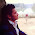It's working for every test case.....
I think you should again check your output....

2.yes its working perfectly

2.the program has stopped working to insert the elements 1,2,3,4,5,6,7 and 8 in that sequence.

3.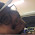the program has stopped working to insert the elements 1,2,3,4,5,6,7 and 8 in that sequence.

4.This comment has been removed by the author.

5.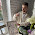There is a mistake. In routine void RBtree::delfix(node *p) there is the next code:
if(s->right->color=='b')
{
s->left->color=='b';
...
== is wrong!

1.what is the right to it ??

6."if(y->color=='b') delfix(q);" in del() is wrong, "&& q != NULL" is lost.

The method "insertfix()" is wrong. logic of the code is not true and leads to endless loop.
Here is correct:
void RBtree::insertfix(node *z)
{
if (z->parent != NULL && z->parent->parent != NULL)
{
while (z != NULL && z->parent != NULL &&
z->parent->parent != NULL && !z->parent->color == 'b')
// ass long as color is not black, thus red
{
if (z->parent == z->parent->parent->left)
{
node *y = z->parent->parent->right;
if (y != NULL && y->color == 'r')
{
z->parent->color = 'b';
y->color = 'b';
z->parent->parent->color = 'r';
z = z->parent->parent;
}
else if (z == z->parent->right)
{
z = z->parent;
leftrotate(z);
}
z->parent->color = 'b';
z->parent->parent->color = 'r';
rightrotate(z->parent->parent);

}
else
{

node *y = z->parent->parent->left; // left instead of right
if (y != NULL && y->color == 'r') // is red?
{
z->parent->color = 'b'; // color = black
y->color = 'b'; // color = black
z->parent->parent->color = 'r'; // color = red
z = z->parent->parent;
}
else
{
if (z == z->parent->left) // left instead of right
{
z = z->parent;
rightrotate(z);
}
z->parent->color = 'b'; // color is black
z->parent->parent->color = 'r'; // color is red
leftrotate(z->parent->parent);
}
}
}
}
}

1.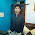This comment has been removed by the author.

2.Awesome .... Thanks a lot :)

3.Using your method makes program crush while youre trying to insert root->left->left element

4.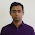Try to insert 10,5,3. Then print the tree and see what it looks like. @Ivan

7.the program has stopped working to insert the elements 1,2,3,4,5,6,7 and 8 in that sequence.

8.Ivan, can you post the code for deletion?
thanks

9.Why does the insertion not go beyond 8 elements?

10.Insertfix is an infinite loop.

When u->color != 'r' nothing is done, so the code will loop. Easy to fix knowing this is what is causing it.

1.may you tell me who is the mistake? thanks ;)

11.What are the variables q and p? I'm confused on what they stand for througout the code.

12.This comment has been removed by the author.

13.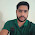program not entering value more than 7

14.Hi Team, It's not working for input = 1,2,3,4,5,6

15.Also there is an error with the fix that when it replaces the root, the new root maintains a link to its previous parent.

Needs to check if parent that node is rotating with if root then change its parent link to nullptr or null.

16.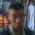cám ơn bạn nhiều lắm.